# Raffle

There are 200 draws in the raffle, but only 20 of them win. What is the probability of at least 4 winnings for a group of people who have bought 5 tickets together?

Correct result:

p =  30.78712 %

#### Solution: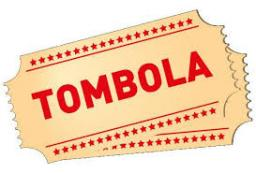We would be pleased if you find an error in the word problem, spelling mistakes, or inaccuracies and send it to us. Thank you!

Showing 1 comment:Math student
it rly helpedTips to related online calculators
Would you like to compute count of combinations?

## Next similar math problems:

• SalamiHow many ways can we choose 5 pcs of salami if we have 6 types of salami for 10 pieces and one type for 4 pieces?
• Hazard game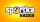In the Sportka hazard game, 6 numbers out of 49 are drawn. What is the probability that we will win: a) second prize (we guess 5 numbers correctly) b) the third prize (we guess 4 numbers correctly)?
• Math logicThere are 20 children in the group, each two children have a different name. Alena and John are among them. How many ways can we choose 8 children to be among the selected A) was John B) was John and Alena C) at least one was Alena, John D) maximum one wa
• Chambers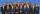The decision-making committee consists of three people. In order for the commission's decision to be valid, at least two members must vote in the same way. It is not possible not to vote in the commission, everyone only votes yes or no. We assume that the
• Boys and girlsThere are eight boys and nine girls in the class. There were six children on the trip from this class. What is the probability that left a) only boys b) just two boys
• Tournament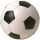How many matches will be played in a football tournament in which there are two groups of 5 teams if one match is played in groups with each other and the group winners play a match for the overall winner of the tournament?
• ClassroomOf the 26 pupils in the classroom, 12 boys and 14 girls, four representatives are picked to the odds of being: a) all the girls b) three girls and one boy c) there will be at least two boys
• DivideHow many different ways can three people divide 7 pears and 5 apples?
• WordWhat is the probability that a random word composed of chars E, Y, G, E, R, O, M, T will be the GEOMETRY?
• Dices throwsWhat is the probability that the two throws of the dice: a) Six falls even once b) Six will fall at least once
• Boys and girlsThere are 11 boys and 18 girls in the classroom. Three pupils will answer. What is the probability that two boys will be among them?
• RectanglesHow many rectangles with area 8713 cm2 whose sides is natural numbers are?
• Pairs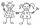From the five girls and four boys teachers have to choose one pair of boy and girl. A) How many such pairs of (M + F)? B) How many pairs where only boys (M + M)? C) How many are all possible pairs?
• Sum or productWhat is the probability that two dice fall will have the sum 7 or product 12?
• Peaches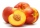There are 20 peaches in the pocket. 3 peaches are rotten. What is the probability that one of the randomly picked two peaches will be just one rotten?There are 15 boys and 12 girls at the graduation party. Determine how many four couples can be selected.How many three-digit numbers are from the numbers 0 2 4 6 8 (with/without repetition)?